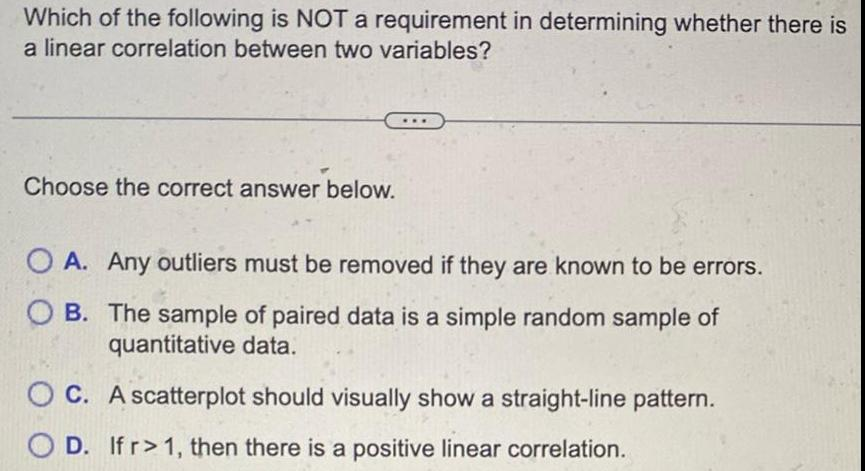Question:

# Which of the following is NOT a requirement in determining

Last updated: 11/19/2023Which of the following is NOT a requirement in determining whether there is a linear correlation between two variables Choose the correct answer below OA Any outliers must be removed if they are known to be errors OB The sample of paired data is a simple random sample of quantitative data OC A scatterplot should visually show a straight line pattern D If r 1 then there is a positive linear correlation